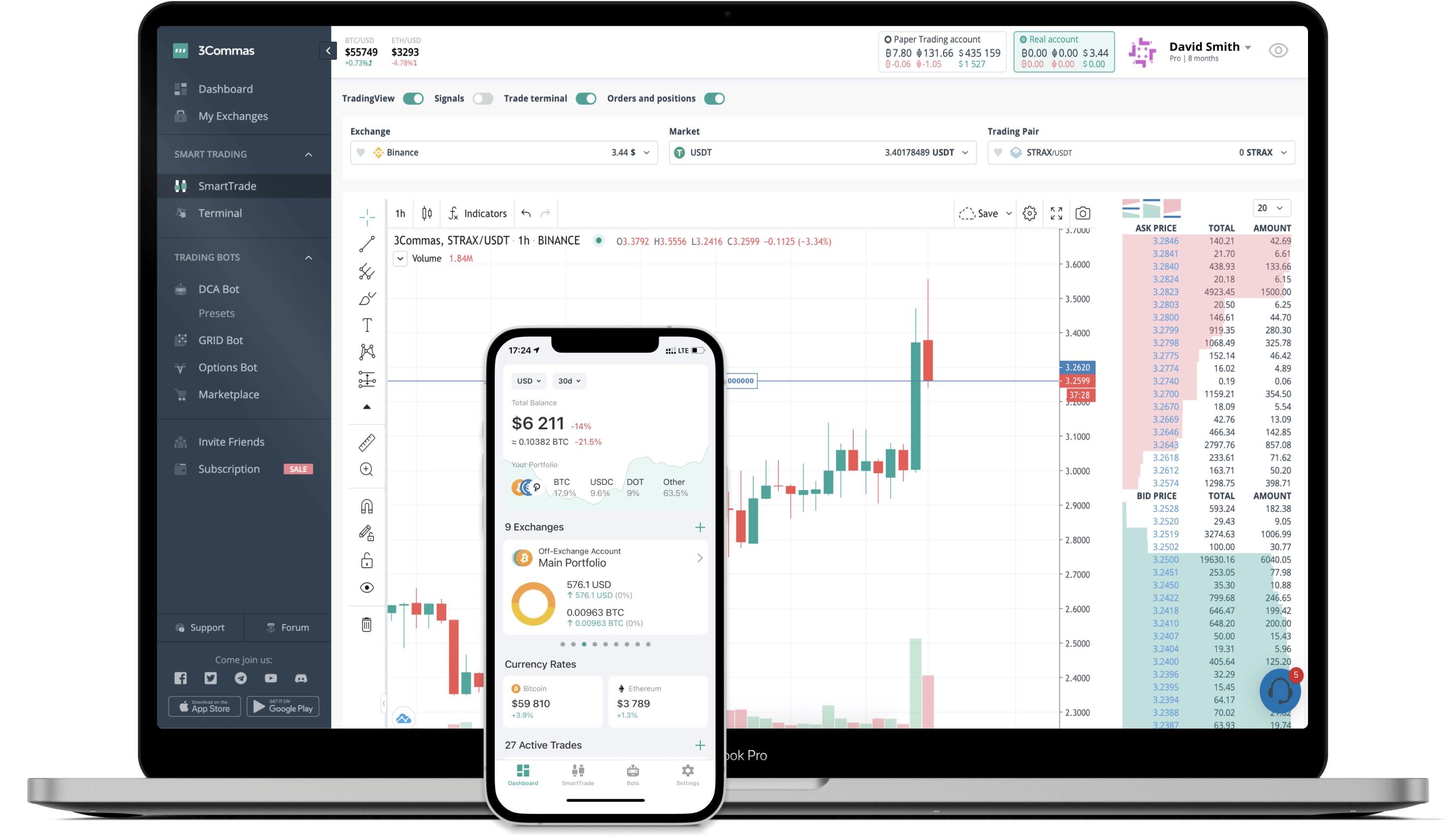ANGLE to JPY Converter

ANGLE
PriceJPY 76.98-16.45%
VolumeJPY 159,196,830
How it works

The 3Commas currency calculator allows you to convert a currency from ANGLE (ANGLE) to Japanese yen (JPY) in just a few clicks at live exchange rates.

Simply enter the amount of ANGLE you wish to convert to JPY and the conversion amount automatically populates. You can also use our Prices Calculator Table to calculate how much your currency is worth in other denominations, i.e. .1 ANGLE, .5 ANGLE, 1 ANGLE, 5 ANGLE, or even 10 ANGLE.

ANGLE to JPY Exchange Calculator

The chart displays ANGLE price changes in JPY.

The ultimate tools for crypto traders to maximize trading profits while minimizing risk and loss.Latest ANGLE to JPY price calculator

ANGLE market price is updated every three minutes and is automatically displayed in JPY. Below are the most popular denominations to convert to JPY.

ANGLE
Japanese yen
0.01
ANGLE
0.77
JPY
0.1
ANGLE
7.70
JPY
1
ANGLE
76.98
JPY
2
ANGLE
153.96
JPY
3
ANGLE
230.94
JPY
5
ANGLE
384.90
JPY
10
ANGLE
769.80
JPY
25
ANGLE
1924.50
JPY
50
ANGLE
3849.00
JPY
100
ANGLE
7698.00
JPY
250
ANGLE
19245.00
JPY
500
ANGLE
38490.00
JPY
1000
ANGLE
76980.00
JPY
2500
ANGLE
192450.00
JPY
Japanese yen
ANGLE
0.01
JPY
0.00012990
ANGLE
0.1
JPY
0.00129904
ANGLE
1
JPY
0.01299039
ANGLE
2
JPY
0.02598077
ANGLE
3
JPY
0.03897116
ANGLE
5
JPY
0.06495194
ANGLE
10
JPY
0.12990387
ANGLE
25
JPY
0.32475968
ANGLE
50
JPY
0.64951936
ANGLE
100
JPY
1.29903871
ANGLE
250
JPY
3.24759678
ANGLE
500
JPY
6.49519356
ANGLE
1000
JPY
12.99038711
ANGLE
2500
JPY
32.47596778
ANGLE

Top Currencies

The price of ANGLE in other fiat and cryptocurrencies is based on the corresponding exchange rate of those currencies to the Japanese yen.

FAQ

How much is 1 ANGLE in Japanese yen?

ANGLE price in JPY is constantly changing.

At this moment, 1 ANGLE equals 76.98 JPY

How do I calculate the conversion from ANGLE to JPY?

The 3Commas ANGLE Calculator allows you to easily calculate the conversion price of ANGLE to JPY by simply entering the amount of ANGLE in the corresponding field and will automatically convert the value in Japanese yen (JPY).

You can also use our ANGLE price table above to check the latest ANGLE price in major fiat and crypto currencies.

How can I convert ANGLE to JPY?

The most common way of converting ANGLE to JPY is by using a Crypto Exchange or a P2P (person-to-person) exchange platform like LocalBitcoins, etc.